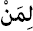Quiz: Do you know how to make questions in Arabic?

Asking questions is essential part in any conversations, and Arabic world is not exception. There are 12 the most common words to use for making up questions in STANDARD Arabic language. These are:(= Ayna) where(= Man) who(= Mata) when(= Maza) what (usually after it, comes a verb)(= Ma) what (usually after it, comes a noun/pronoun)(= Lemaza) why(= Leman) for who / for whom

Examples:
أين المِفْتَاح؟ (= ayna al-muftah?) where is the key?
مَن هُنَا؟ (= man huna?) who is here?
مَتَى نَأْكُل؟ (= mata na'kul?) when do we eat?
مَاذَا تَفْعَل؟ (= maza tafal?) what are you doing?
مَا لَوْنُكَ المُفَضَّل؟ (= ma huwa lawnuka al-mufadhal?) what is your favorite color?
لِمَاذَا تَأَخَّرْت؟ (= lemaza ta'khart?) why you are late?
هَل أَنْتَ سَعِيد؟ (= hal anta saeed?) are you happy?
كَيفَ عَرَفْتَ الحَل؟ (= kaifa arafta al-hal?) how did you know the answer?
بِكَم هذا القَلَم؟ (= bekam al-qalam?) how much is this pen?
أي فِلْم سَنُشَاهِد؟ (= ayyu film nushahed?) which film shill we watch?
بِمَاذَا تَكْتُب؟ (= bemaza taktub?) how do you write? by what?
لِمَن هَذِا المِعْطَف؟ (= leman haza al-meataf?) for whom is this jacket?

Now, take this quiz to test your understanding about using question words in Arabic:

Notes:
• بكم is actually come from the word --> كَم (= kam) which is used to ask for a number (ex: price, age, group members..). Examples to use كم:
بِـكَم الحَقِيبة؟ (= be-kam al-haqeeba?) how much is the bag?
كَم عُمْرُك؟ (= kam umruk?) how old are you?
كَم طَالبًا في الفصل؟ (= kam taleban fee al-fasl?) how many students in the class?
• There are other questions tool that are using in Arabic language:
أ (= A') for Yes/No question, أنّى (= Anna) means How and أيان (= Ayyana) means When.
since in general these are not often used, we didn't put examples for them. If you have any question, put it in comments below and I'll be happy to answer.
• All the questions tools are used in the beginning of the question but in some dialects it is different sometimes and may used in the end of the question. For example in egyptian dialect:
عَامِل إيه؟ (= Amel eih?) what are you doing. إيه (= eih) is used in the end of the question.

All quizzes in EVERYDAY ARABIC
Quiz: introduce yourself in Arabic
Quiz: Prepositions in Arabic

Sharing is Caring »»

 Tweet

2 التعليقات:

1.1.